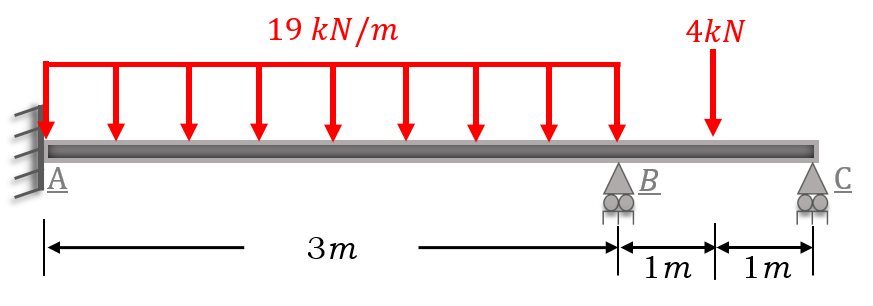Beam Analysis by the Matrix Method of Rigidity | Excel ©HM.Beams V.1

Example 1: Analyze the beam shown in the figure that is subjected to a distributed load and a point force, use the matrix method of stiffness. EI = Constant Solution: a) Define the degrees of freedom and orientation of the element. Note: The beam is not subjected to axial load, therefore two degrees of freedom …